Question

# Theorem 3.14 Let (neN aand EneN be sequences in R. Let be in R# and suppose that x" → x, y, → oo,...

Finish the proof of Theorem 3.14.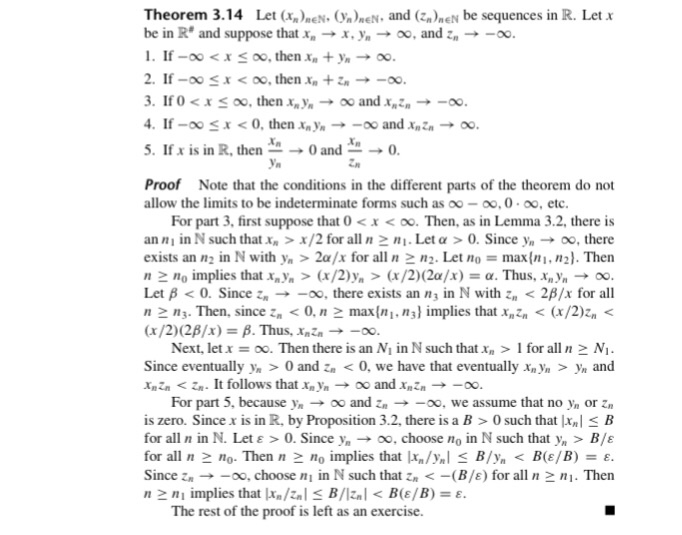Theorem 3.14 Let (neN aand EneN be sequences in R. Let be in R# and suppose that x" → x, y, → oo, and z" →-oo. . If -oo (x/2)(2a/x): 'Thus, xn yn-+ 00 Let β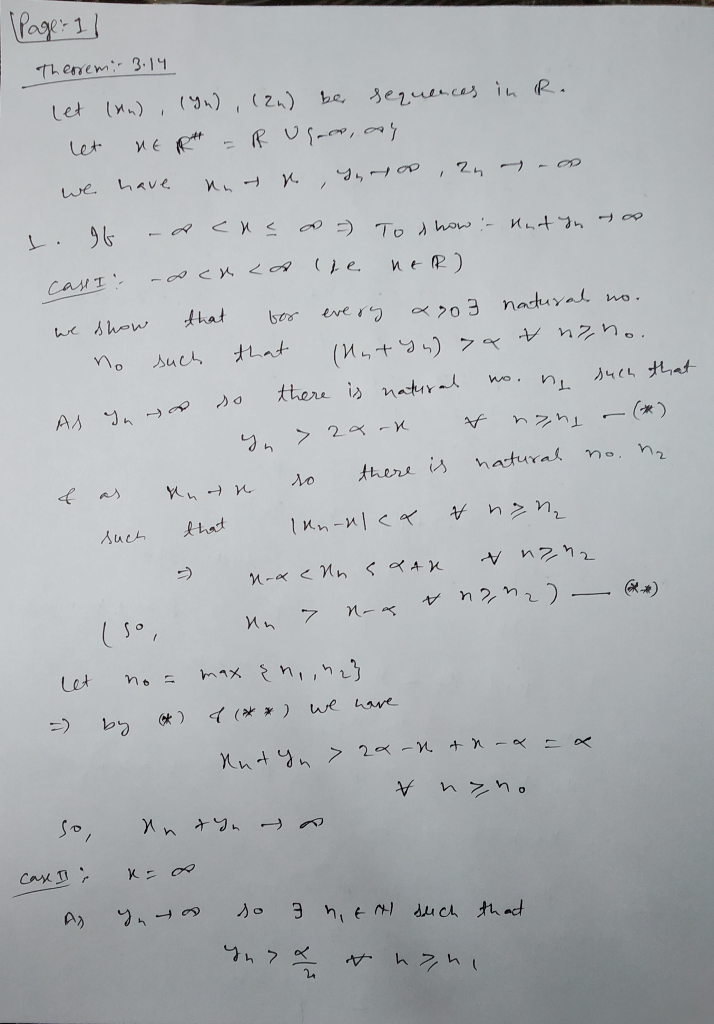1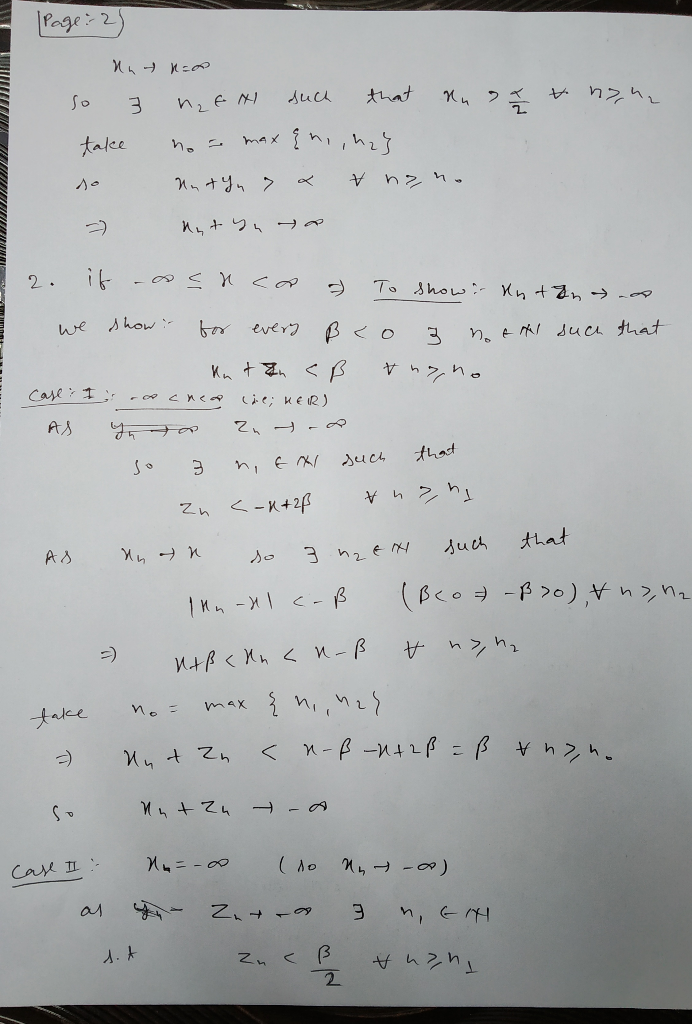2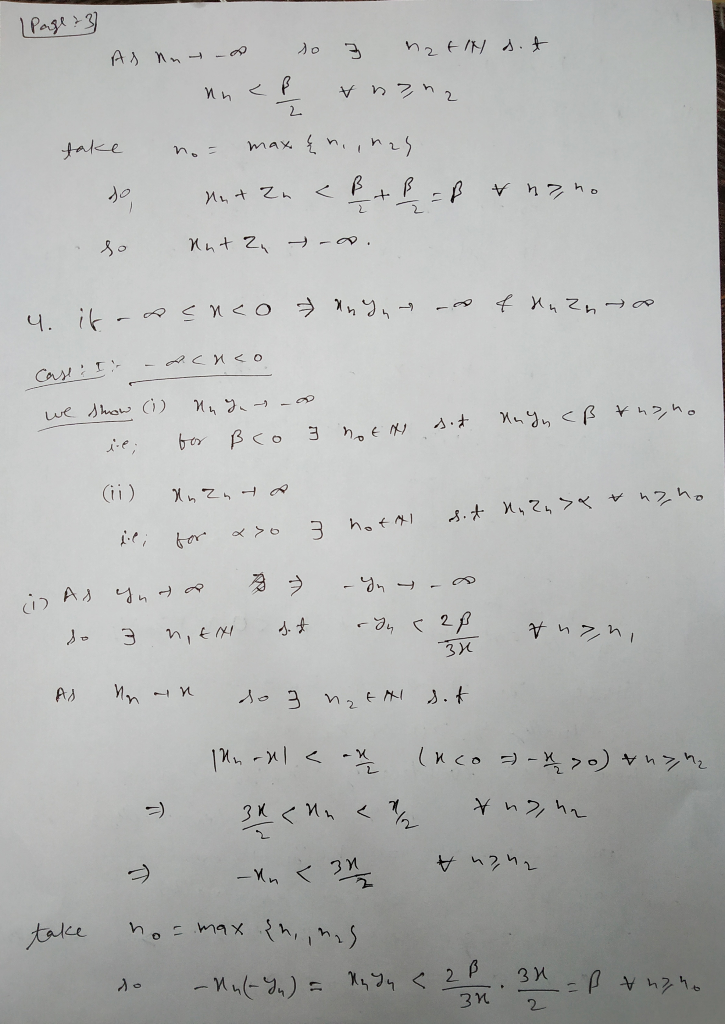3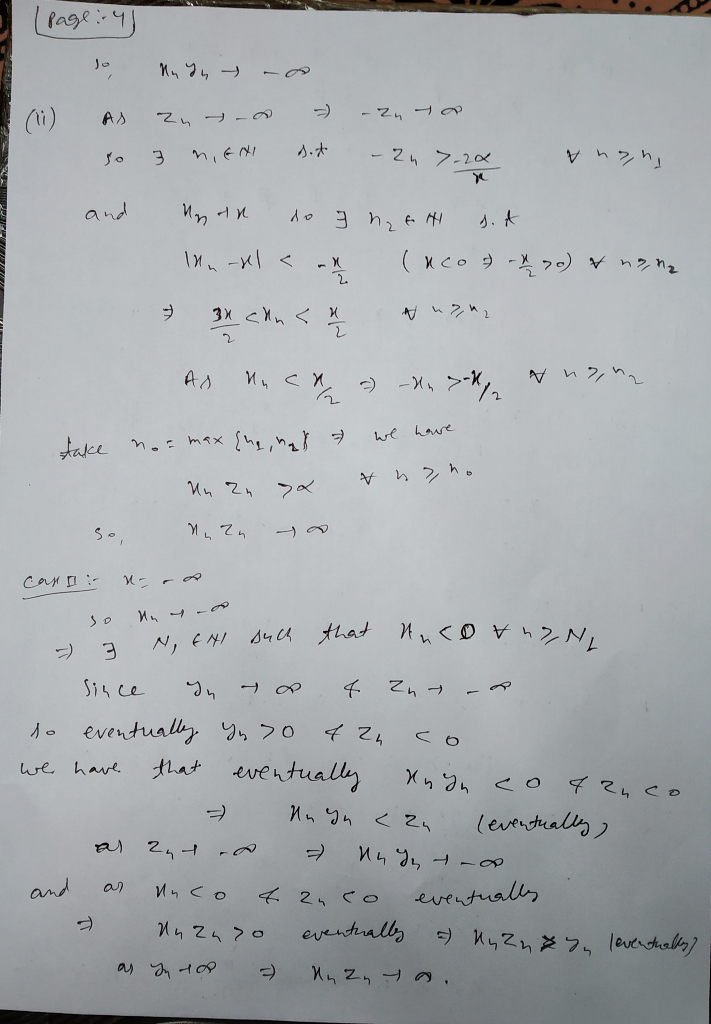4

#### Earn Coins

Coins can be redeemed for fabulous gifts.

Similar Homework Help Questions
• ### 2. Let (En)acy be a sequence in R (a) Show that xn → oo if and only if-An →-oo. (b) If xn > 0 for...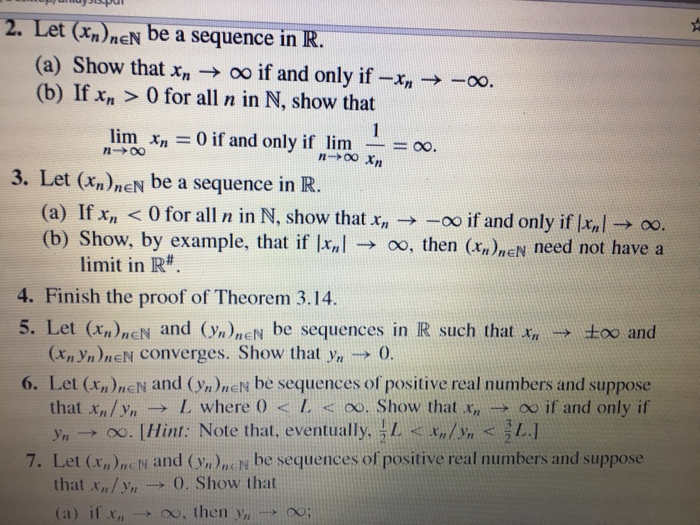#s 2, 3, 6 2. Let (En)acy be a sequence in R (a) Show that xn → oo if and only if-An →-oo. (b) If xn > 0 for all n in N, show that linnAn = 0 if and only if lim-= oo. 3. Let ()nEN be a sequence in R. (a) If x <0 for all n in N, show that - -oo if and only if xl 0o. (b) Show, by example, that if kal → oo,...

• ### Problem 2 Let {xn) , (yn) and {zn^ be three number sequences and suppose that for...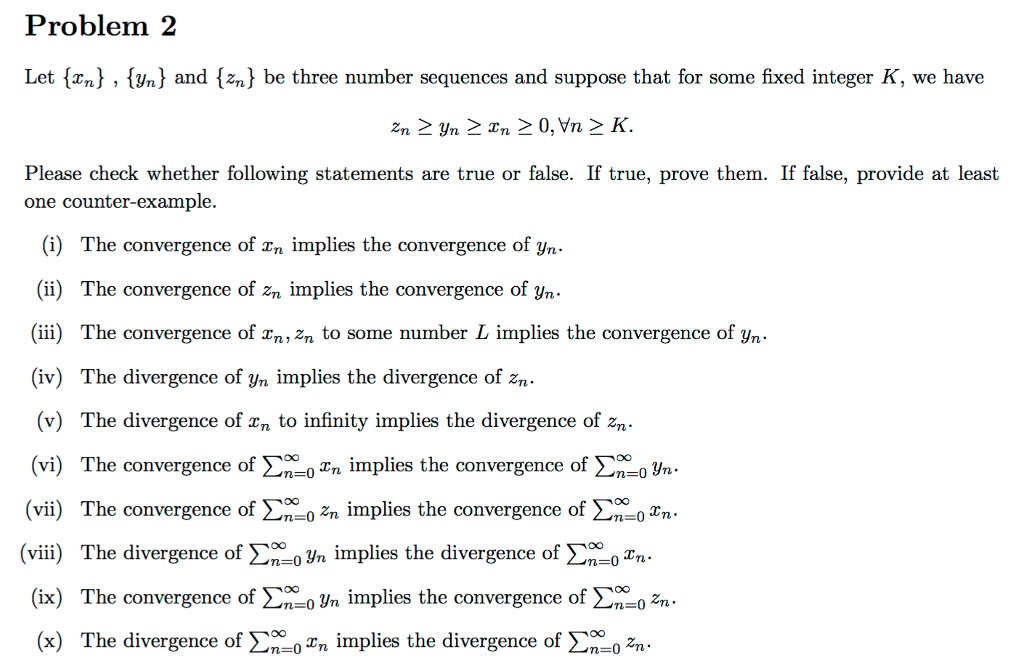Problem 2 Let {xn) , (yn) and {zn^ be three number sequences and suppose that for some fixed integer K, we have Please check whether following statements are true or false. If true, prove them. If false, provide at least one counter-example. (i) The convergence of rn implies the convergence of yn (ii) The convergence of zn implies the convergence of yn. (ii) The convergence of n, zn to some number L implies the convergence of yn (iv) The divergence...

• ### 2. Let {xn}nEN be a sequence in R converging to x 0. Show that the sequence R. Assume that x 0 an...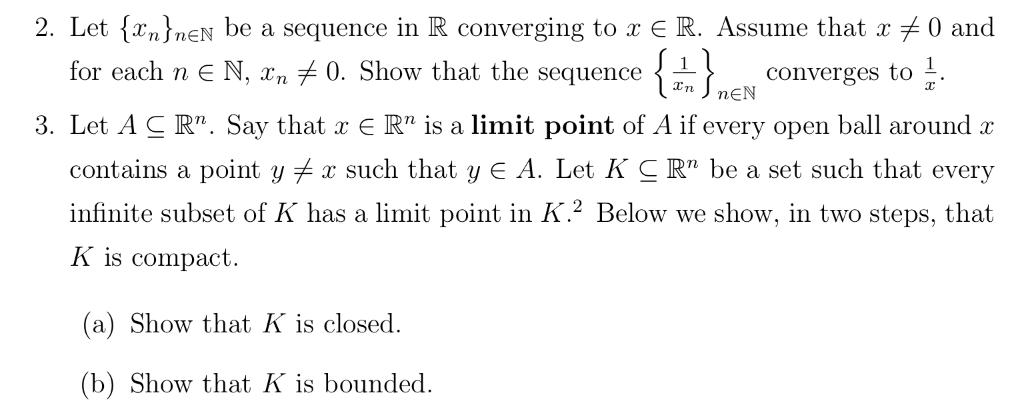2. Let {xn}nEN be a sequence in R converging to x 0. Show that the sequence R. Assume that x 0 and for each n є N, xn converges to 1. 3. Let A C R". Say that x E Rn is a limit point of A if every open ball around x contains a point y x such that y E A. Let K c Rn be a set such that every infinite subset of K has a limit...

• ### 2. Let f(x,y) = e-r-u, 0 < x < oo, 0 < y < oo, zero...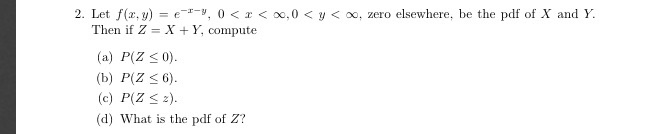2. Let f(x,y) = e-r-u, 0 < x < oo, 0 < y < oo, zero elsewhere, be the pdf of X and Y. Then if Z = X + Y, compute (a) P(Z 0). (b) P(Z 6) (c) P(Z 2) (d) What is the pdf of Z?

• ### 1. Let {y,)%, be a sequence of random variables, and let Y be a random variable on the same sample space. Let A(E) be the event that Y - Y e. It can be shown that a sufficient condition for Y, to con...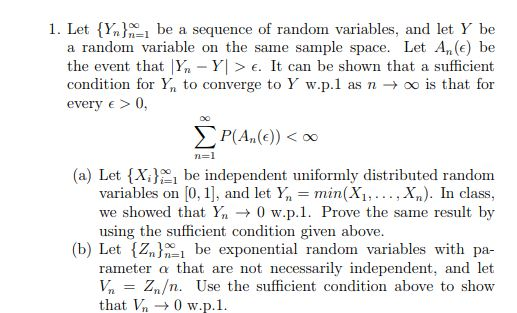1. Let {y,)%, be a sequence of random variables, and let Y be a random variable on the same sample space. Let A(E) be the event that Y - Y e. It can be shown that a sufficient condition for Y, to converge to Y w.p.1 as n → oo is that for every e0, (a) Let {Xbe independent uniformly distributed random variables on [0, 1] , and let Yn = min (X), , X,). In class, we showed that...

• ### 253 42. Suppose that Xn B(n, p), and let p X/n. The CLT implies that se...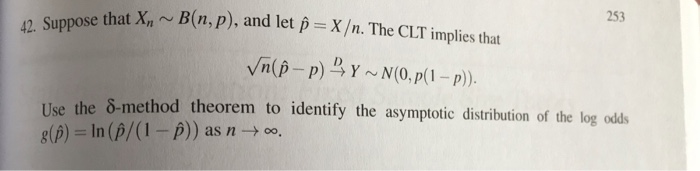253 42. Suppose that Xn B(n, p), and let p X/n. The CLT implies that se the 8-method theorem to identify the asymptotic distribution of the log odss g(β) = ln (p/0-p)) as n → 00,

• ### Please do 10 & 11 Use Intermediate Value Theorem lial p(x) = x4 +7x = 9...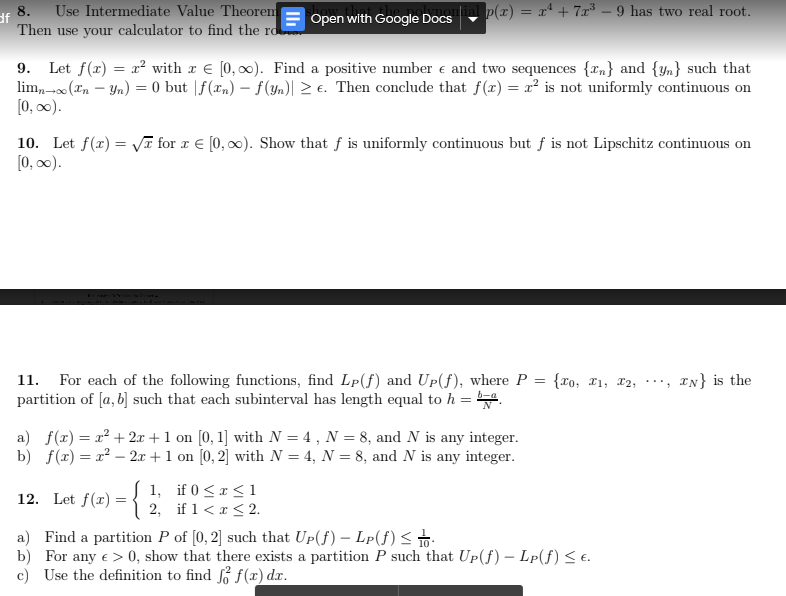Please do 10 & 11 Use Intermediate Value Theorem lial p(x) = x4 +7x = 9 has two real root. 8. df Open with Google Docs Then use your calculator to find the ro 9 Let f(z)2with € [0, 00). Find a positive mumber e and two sequences {xn} and {yn} such that lim-(nn) = 0 but |f(xn)- f(Yn)| 2 e. Then conclude that f(x) = x2 is not uniformly continuous on [0, ao) [0, oo). Show that f is...

• ### Theorem 10.1.15 (Chain rule). Let X, Y be subsets of R, let xo e X be...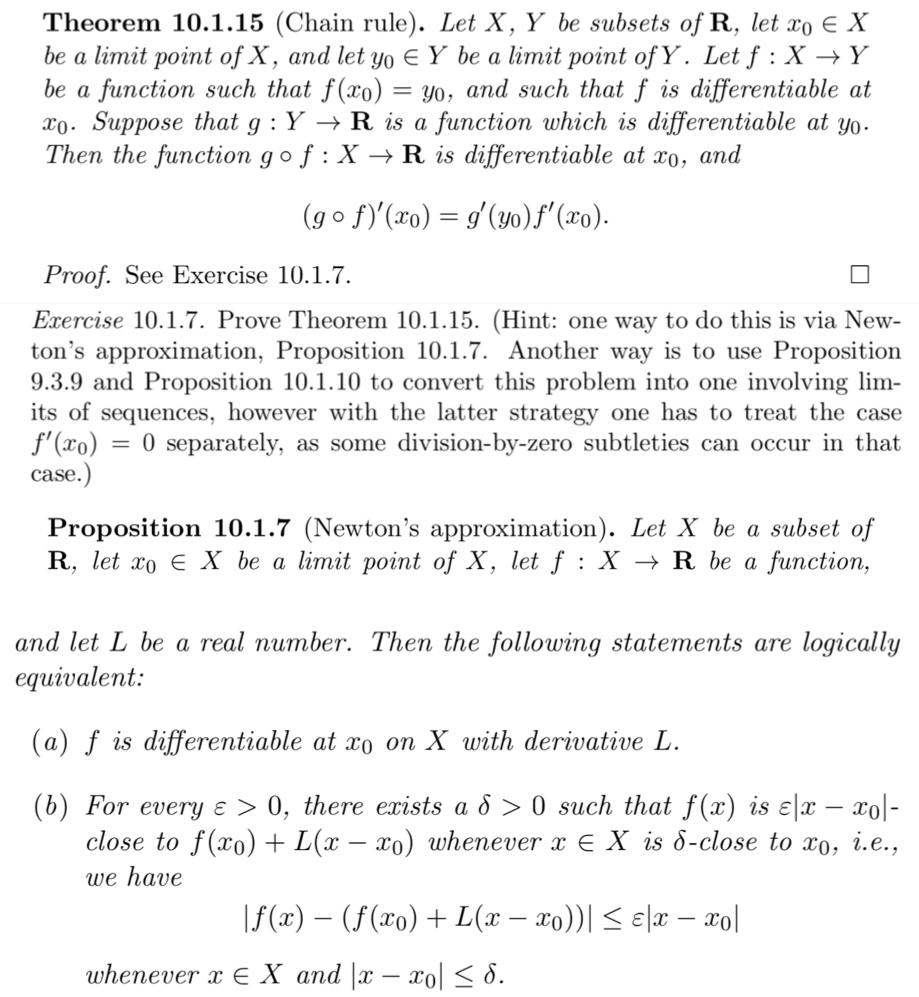Theorem 10.1.15 (Chain rule). Let X, Y be subsets of R, let xo e X be a limit point of X, and let yo e Y be a limit point of Y. Let f : X+Y be a function such that f(xo) = yo, and such that f is differentiable at Xo. Suppose that g:Y + R is a function which is differentiable at yo. Then the function gof:X + R is differentiable at xo, and .. (gºf)'(xo) = g'(yo)...

• ### Theorem 2. Let E be an open subset of R² and suppose that fe C'(E). Let...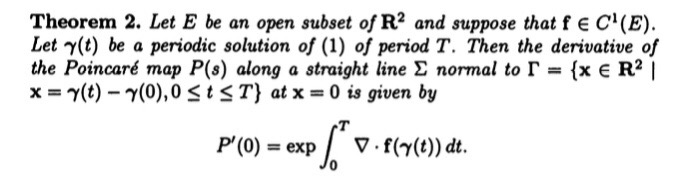Theorem 2. Let E be an open subset of R² and suppose that fe C'(E). Let y(t) be a periodic solution of (1) of period T. Then the derivative of the Poincaré map P(8) along a straight line normal to r = {x E R x = y(t) - (0),O SE ST} at x = 0 is given by T P(0) = exp V. f(y(t)) dt. 4. Show that the system • = -y + (1 – 22 - y2)2...

• ### 5. Let {xn} and {yn} be sequences of real numbers such that x1 = 2 and...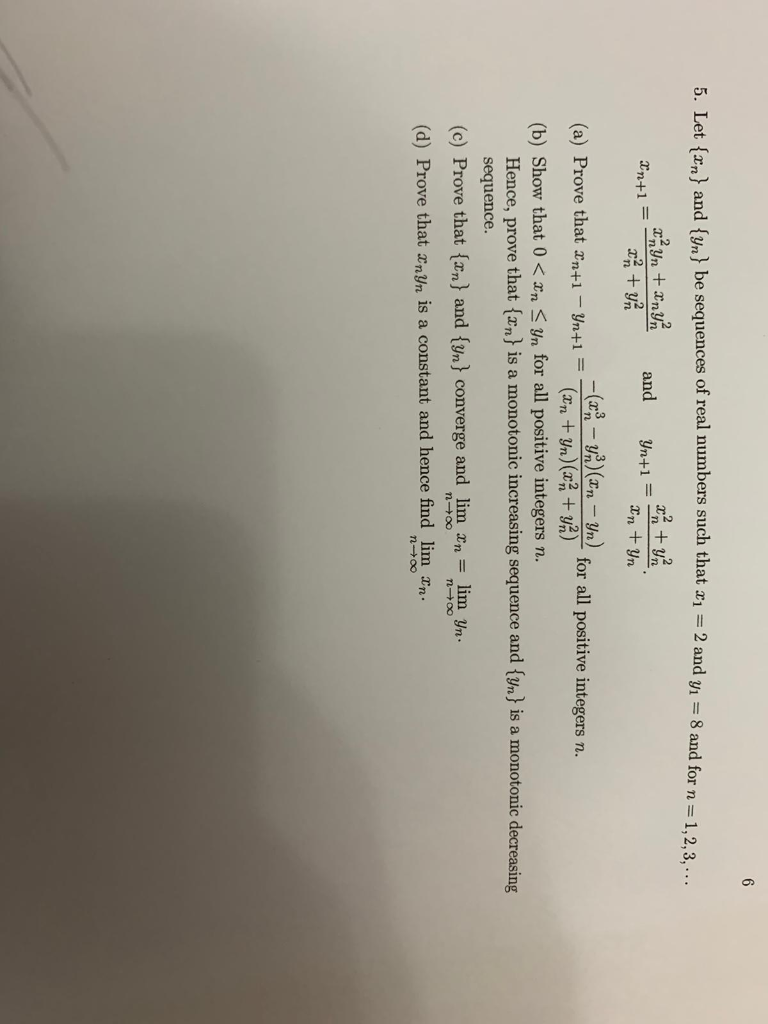5. Let {xn} and {yn} be sequences of real numbers such that x1 = 2 and y1 = 8 and for n = 1,2,3,··· x2nyn + xnyn2 x2n + yn2 xn+1 = x2 + y2 and yn+1 = x + y . nn nn (a) Prove that xn+1 − yn+1 = −(x3n − yn3 )(xn − yn) for all positive integers n. (xn +yn)(x2n +yn2) (b) Show that 0 < xn ≤ yn for all positive integers n. Hence, prove...